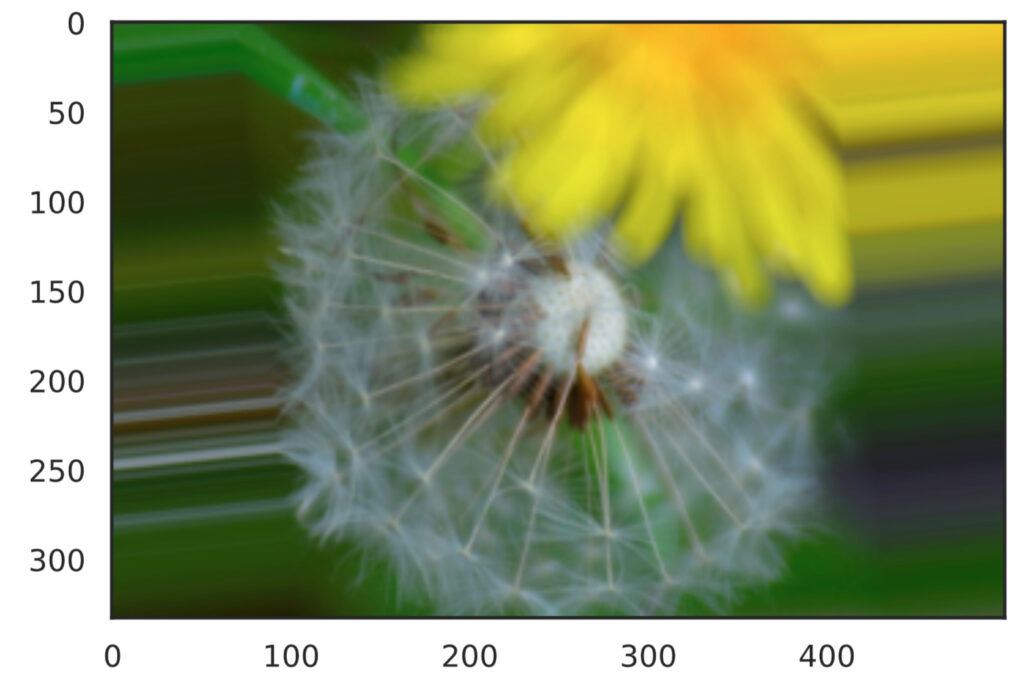# 【ImageDataGenerator】Data Augmentation Of Training Images In Keras

## Introduction

Data Augmentation is a useful technique for deep learning image recognition when there is not enough training image data. This technique creates new training image data by artificially processing and combining data. Image processing methods generally include image cropping, inversion, and transformations such as changes in brightness and saturation.

This article describes how to use keras’ ImageDataGenerator to extend data.

The code described in this article can be tried at ▼

## Try Data Augmentation with ImageDataGenerator

##### Importing libraries and images

First, import the necessary libraries and prepare the images to be converted. In this case, we will try this image.

import numpy as np
import matplotlib.pyplot as plt
import tensorflow as tf
from tensorflow import keras
from tensorflow.keras.preprocessing.image import ImageDataGenerator, load_img, array_to_img

img_path = 'Path of the target image'
target_img = np.array(target_img)
x = target_img.reshape((1,) + target_img.shape)

Here, the ImageDataGenerator class requires a 4-dimensional array in ndarray format, so the last line performs the conversion.

##### Create ImageDataGenerator
# Random rotation in the range [-90°, 90°].
data_generator = ImageDataGenerator(rotation_range=90)
generator = data_generator.flow(x, batch_size=1)
batches = next(generator)
get_img = array_to_img(batches)

plt.imshow(gen_img)
plt.show()

Retrieve and display the transformed image from the ImageDataGenerator. For now, we will apply a 90-degree rotation transformation. There are various other transformations, which will be described later.

The generator of the transformed image is obtained in line 4, and a 4-dimensional array containing the transformed image information is generated in line 6.

When executed, the rotated image is displayed as shown here.▲I was able to rotate and convert the image.
##### Save the converted image

The following arguments can be added to the flow() function to save the converted image.

import os

save_dir = 'output'
os.makedirs(save_dir, exist_ok=True)

data_generator = ImageDataGenerator(rotation_range=90)
generator = data_generator.flow(x, batch_size=1, save_to_dir=save_dir, save_prefix='generated', save_format='png')
batches = next(generator)

## Various conversions

Various image transformations can be performed by changing the parameters passed to ImageDataGenerator. Here we use the following function definition to display four converted images.

def show_gen_img(data_generator):
generator = data_generator.flow(x, batch_size=1)
plt.figure(figsize=(17,8))
for i in range(4):
batches = next(generator)
gen_img = array_to_img(batches)
plt.subplot(1, 4, i+1)
plt.imshow(gen_img)
plt.show()
##### Rotation
# Random rotation in the range [-120°, 120°].
data_generator = ImageDataGenerator(rotation_range=120)
show_gen_img(data_generator)
##### Up and down anti-turning
# Randomly flipped upside down
data_generator = ImageDataGenerator(vertical_flip=True)
show_gen_img(data_generator)
##### Left and Right Reverse Turning
# Random left-right reversal
data_generator = ImageDataGenerator(horizontal_flip=True)
show_gen_img(data_generator)
##### Upper and lower parallel movement
# Random vertical translation within the range of [-0.2*Height, 0.2*Height]
data_generator = ImageDataGenerator(height_shift_range=0.2)
show_gen_img(data_generator)
##### Left and right parallel movement
# Random left/right translation in the range of [-0.2*Width, 0.2*Width]
data_generator = ImageDataGenerator(width_shift_range=0.2)
show_gen_img(data_generator)
##### Shearing
# Random shear in the range of [-20°, 20°]
data_generator = ImageDataGenerator(shear_range=20)
show_gen_img(data_generator)
##### Scaling
# Random scaling in the range [0.3, 1.5]
data_generator = ImageDataGenerator(zoom_range=[0.3, 1.5])
show_gen_img(data_generator)
##### Brightness change
# Randomly change brightness in the range [0.3, 2.0
data_generator = ImageDataGenerator(brightness_range=[0.3, 2.0])
show_gen_img(data_generator)Yukkuri Machine Learning
タイトルとURLをコピーしました